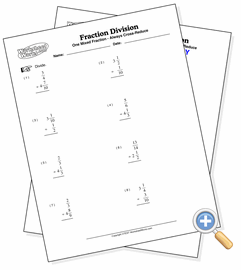# With One Mixed Fraction

## Fraction DivisionDevelop division skills involving simple and mixed fractions

These problems involve the division of a simple fraction into a mixed fraction (or the reverse). This provides the most difficult fraction multiplication problem since it involves converting to improper fractions, inverting the second fraction, cross-reducing, multiplying and finally simplifying in each problem.